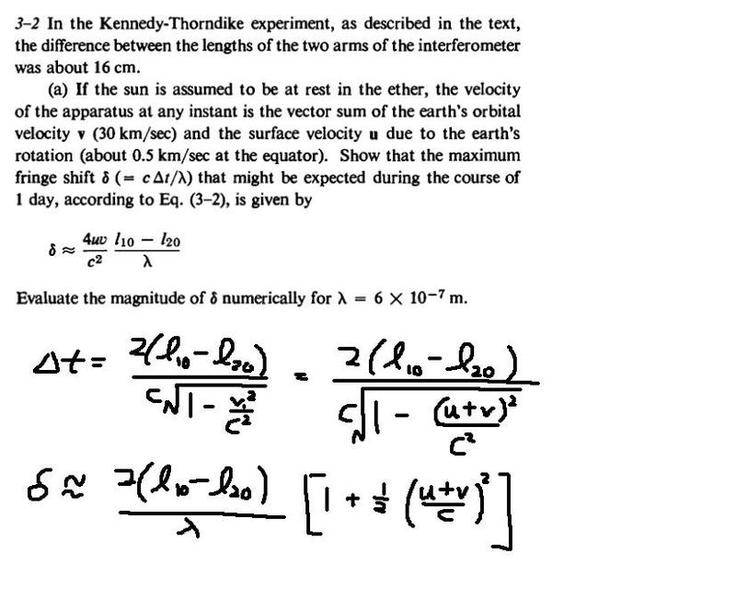# Kennedy-Thorndike Experiment

unscientific

## Homework StatementIn this problem i take the maximum relative velocity of ether wind to be (u + v) where they both complement each other..

## The Attempt at a Solution

Not sure how they ended up with a '4uv' term..

unscientific
Would appreciate any help..

Homework Helper
Gold Member
In this problem i take the maximum relative velocity of ether wind to be (u + v) where they both complement each other..
Good. What would the minimum relative velocity be during the day? δ is the change in Δt(c/λ) in going from maximum to minimum relative velocity.

Last edited:
unscientific
Good. What would the minimum relative velocity be during the day? δ is the change in Δt(c/λ) in going from maximum to minimum relative velocity.

I got very close to the answer, just that my term had a '2uv' instead of a '4uv'..

Homework Helper
Gold Member
Did you calculate Δt for both the maximum and minimum velocity relative to the ether and then take the difference between these two Δt's? Also note the initial factor of 2 in the expression for Δt.

unscientific
Did you calculate Δt for both the maximum and minimum velocity relative to the ether and then take the difference between these two Δt's? Also note the initial factor of 2 in the expression for Δt.

Yes, but there is a factor of (1/2) beside the square...

unscientific
Ah, I see my mistake now..